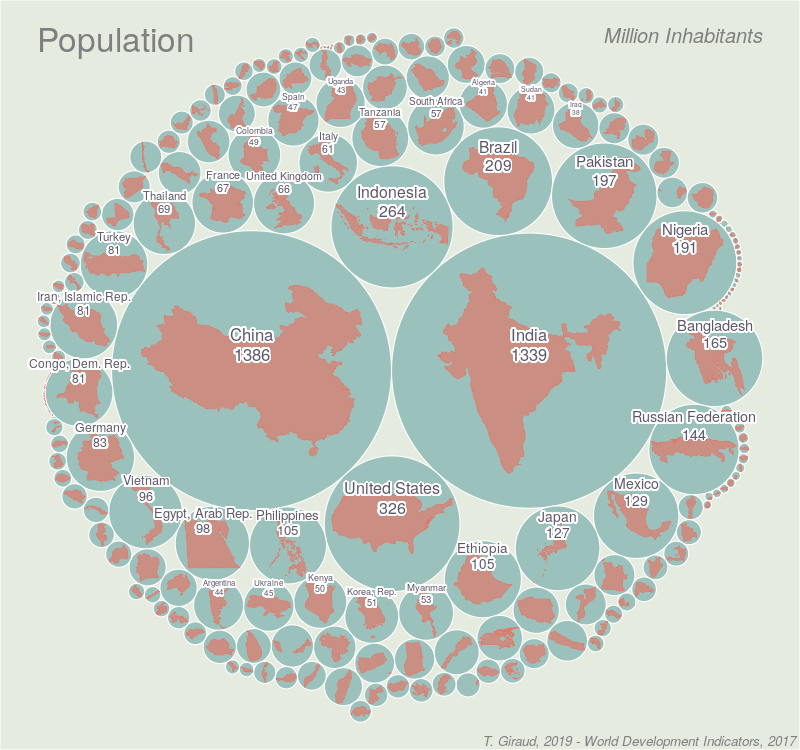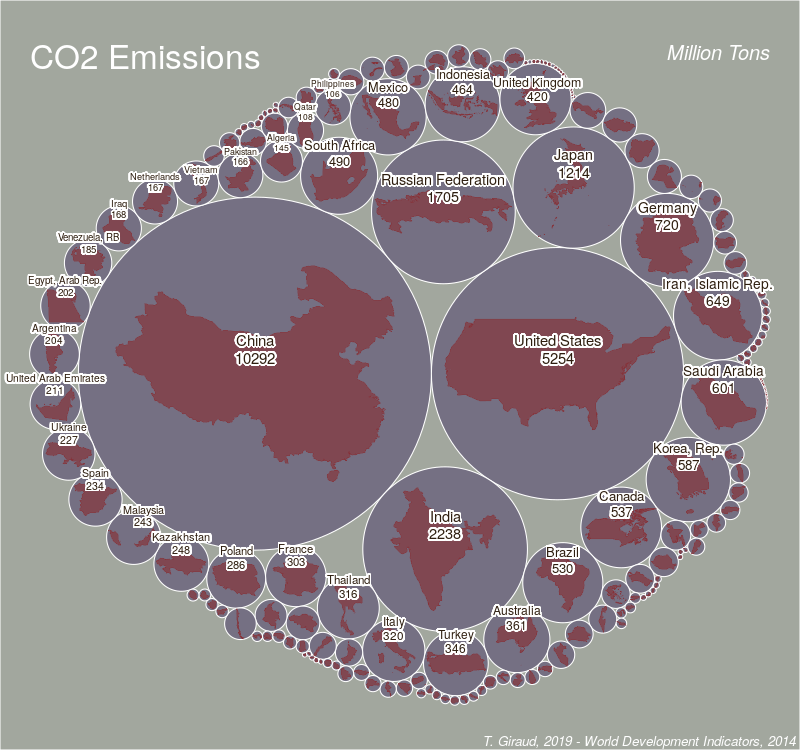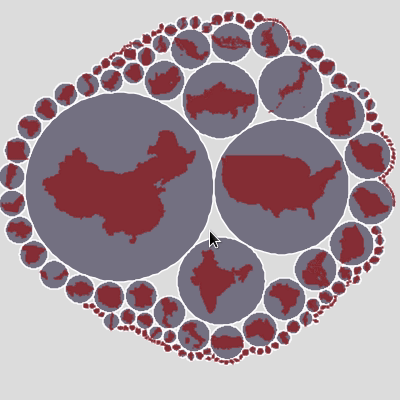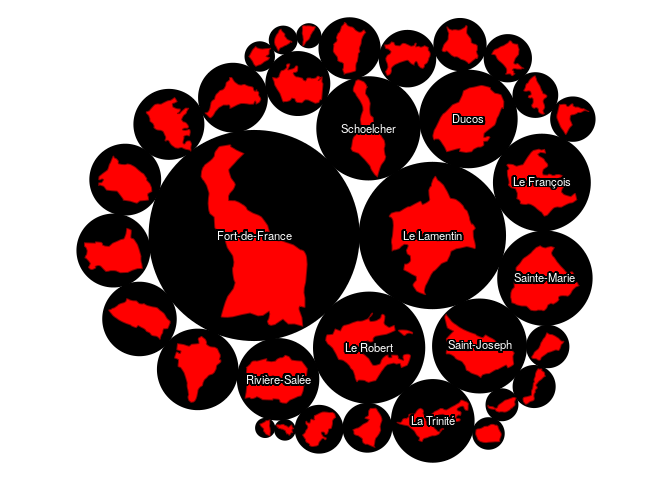# popcircleThis one-function package computes circles with areas scaled to a variable and displays them using a compact layout (higher values in the center, lower values at the periphery). Original polygons are scaled to fit inside these circles (size are roughly proportional, not strictly).## Install

``````library(remotes)
install_github("rCarto/popcircle")
``````

## Example

See this gist for the code used to produce figures on countries population and CO2 emissions.

### Interactive Visualisation

Here is an example of interactive visualisation using `leaflet`.### Typical example

``````library(sf)
library(popcircle)
mtq <- st_read(system.file("gpkg/mtq.gpkg", package="popcircle"))
res <- popcircle(x = mtq, var = "POP")
circles <- res\$circles
shapes <- res\$shapes
par(mar = c(0,0,0,0))
plot(st_geometry(circles), col = "black", border = "black")
plot(st_geometry(shapes), col = "red", add = TRUE, lwd = 1, border = "red4")
if(require(cartography)){
labelLayer(x = circles[1:10,], txt = "LIBGEO", halo = TRUE, overlap = FALSE,
col ="white", bg = "black", r = .15)
}
``````rCarto/popcircle documentation built on May 3, 2019, 8:36 p.m.